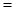Chapter 5, Problem 93IL

Chapter
Section
Textbook Problem

A piece of lead with a mass of 27.3 g was heated to 98.90 °C and then dropped into 15.0 g of water at 22.50 °C. The final temperature was 26.32 °C. Calculate the specific heat capacity of lead from these data.

Interpretation Introduction

Interpretation:

The specific heat capacity of lead has to be calculated.

Concept Introduction:

Heat energy required to raise the temperature of 1g of substance by 1K.Energy gained or lost can be calculated using the below equation.

q=C×m×ΔT

Where, q= energy gained or lost for a given mass of substance (m), C =specific heat Capacity,ΔTchange in temperature.

Explanation

Given mass of the lead is 27.3g

Specific heat capacity of water is 4.184J/gK

Assume qmetal=-qwater

Substitute the values in the equations:

q=C×m×ΔT

27.3g×Cmetal(299.47372.05)K

Still sussing out bartleby?

Check out a sample textbook solution.

See a sample solution

The Solution to Your Study Problems

Bartleby provides explanations to thousands of textbook problems written by our experts, many with advanced degrees!

Get Started

The energy intake recommendation is set at a level predicted to maintain body weight. T F

Nutrition: Concepts and Controversies - Standalone book (MindTap Course List)

Why would you not plot sound waves in the electromagnetic spectrum?

Horizons: Exploring the Universe (MindTap Course List)

Where are protons, electrons, and neutrons found in an atom?

Biology: The Dynamic Science (MindTap Course List)